# Errorbars

Error bars are a simply addition to your graph, utilising their own geometric command `geom_errorbar()`. To add the error bars, we use the following command

``````ggplot(weeds.summarise, aes(x=species, y=mean)) +
geom_bar(stat="identity")+
geom_errorbar(aes(ymin = mean-se, ymax = mean+se))``````This is suprisingly simple. All we do is specify the aesthetic (aes) where we compute our minimum and maximum y values for our bars as our mean column +/- our standard error column.

We can further customise our errorbars through the use of a few arguments. Lets explore those iteratively.

#### Size

``````ggplot(weeds.summarise, aes(x=species, y=mean)) +
geom_bar(stat="identity")+
geom_errorbar(aes(ymin = mean-se, ymax = mean+se), size = 2)``````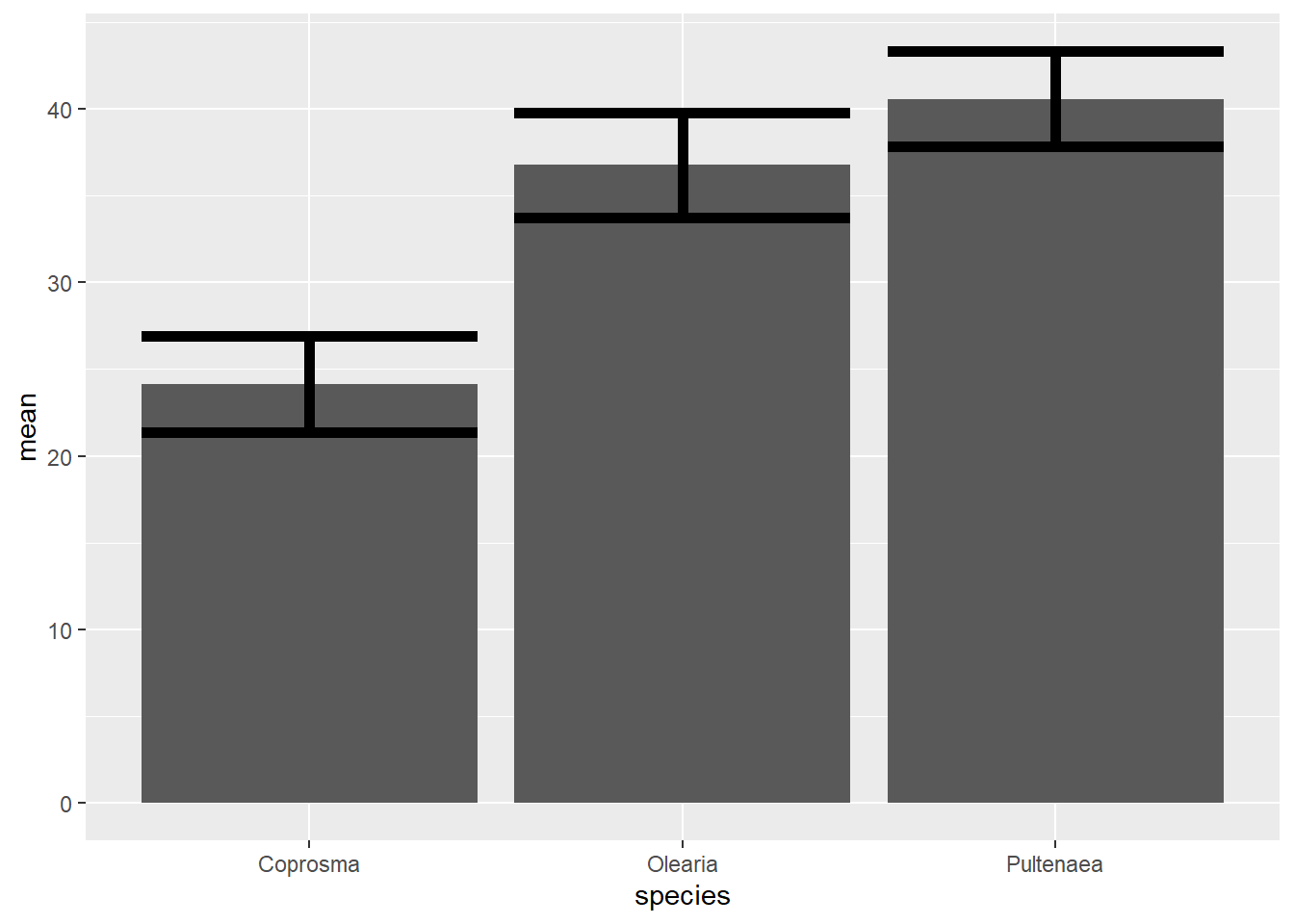The size argument increases the thickness of the errorbars

#### Colour, linetype and transparency

We can also change the colour, linetype, and transparency.

``````ggplot(weeds.summarise, aes(x=species, y=mean)) +
geom_bar(stat="identity")+
geom_errorbar(aes(ymin = mean-se, ymax = mean+se), colour = "red")``````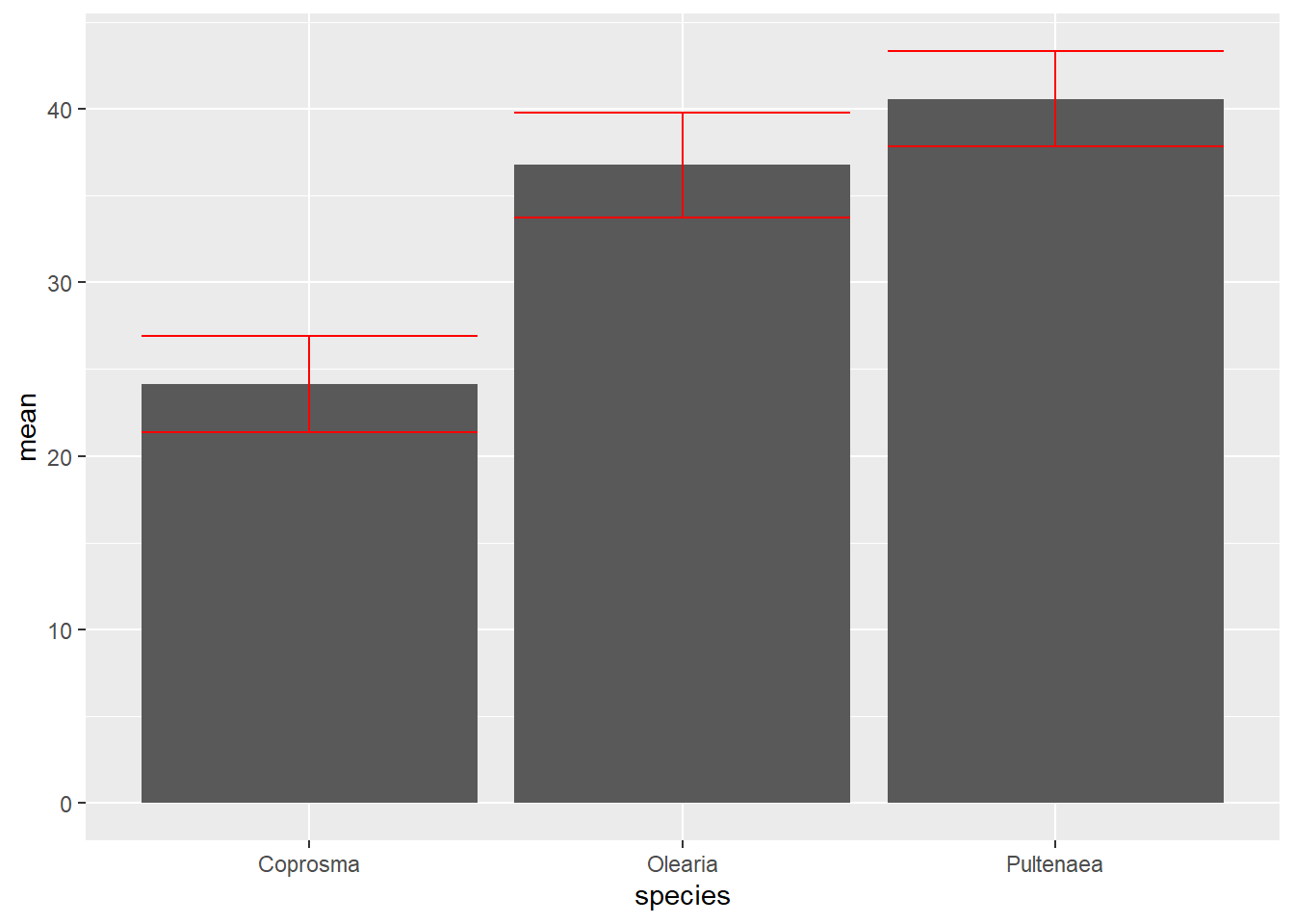Colour is as straightforward as usual, just name a colour.

For linetype, we specify a number between 1-6 that corresponds to R’s built in linetypes.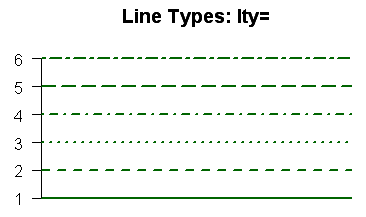``````ggplot(weeds.summarise, aes(x=species, y=mean)) +
geom_bar(stat="identity")+
geom_errorbar(aes(ymin = mean-se, ymax = mean+se), colour = "red", linetype = 2)``````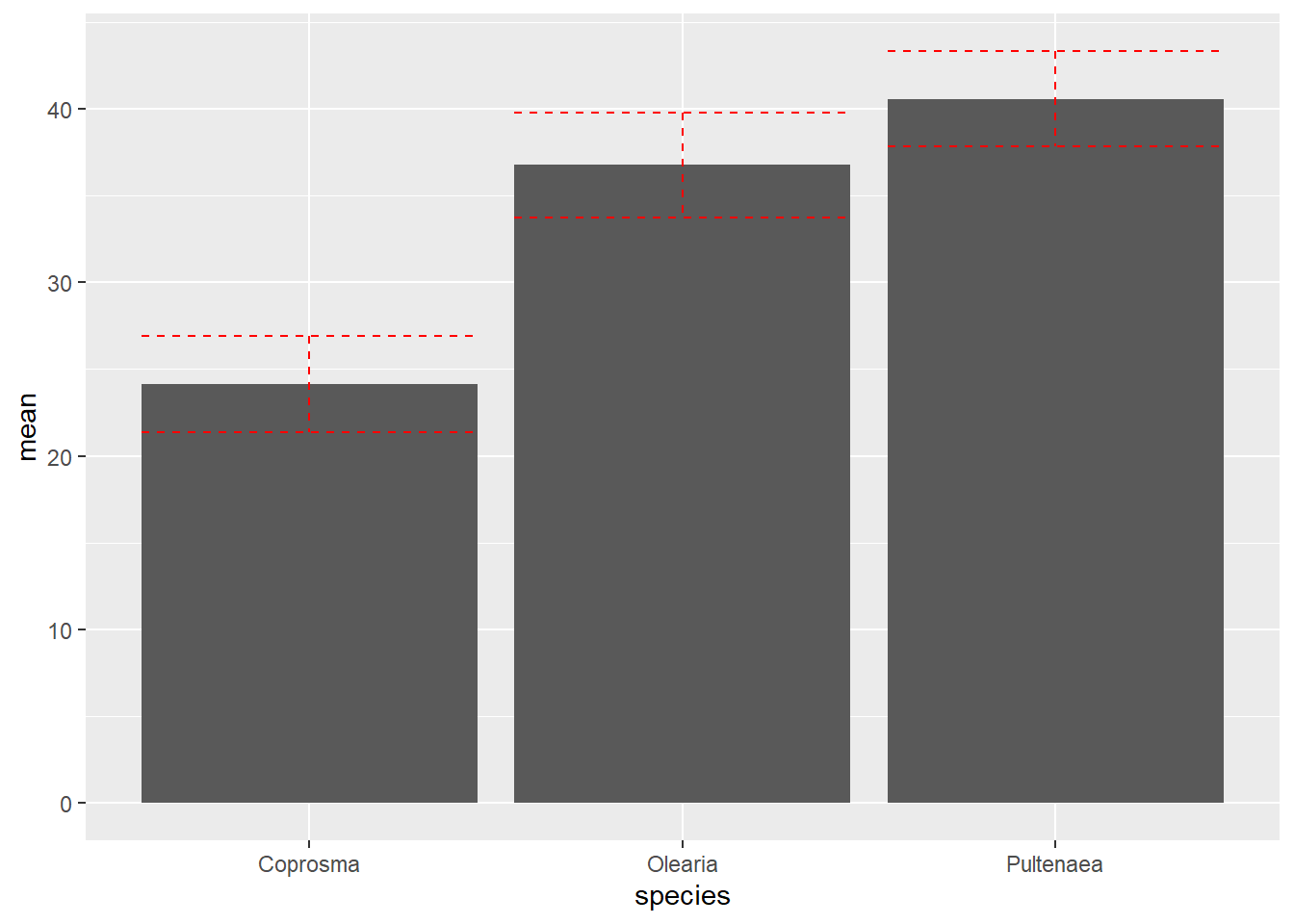Transparency is specified throught the `alpha` argument, giving a number between 0 (transparent) and 1 (solid). It’s pretty pointless for errorbars, but it can be used for many other functions.

``````ggplot(weeds.summarise, aes(x=species, y=mean)) +
geom_bar(stat="identity")+
geom_errorbar(aes(ymin = mean-se, ymax = mean+se), size = 2, alpha = 0.5)``````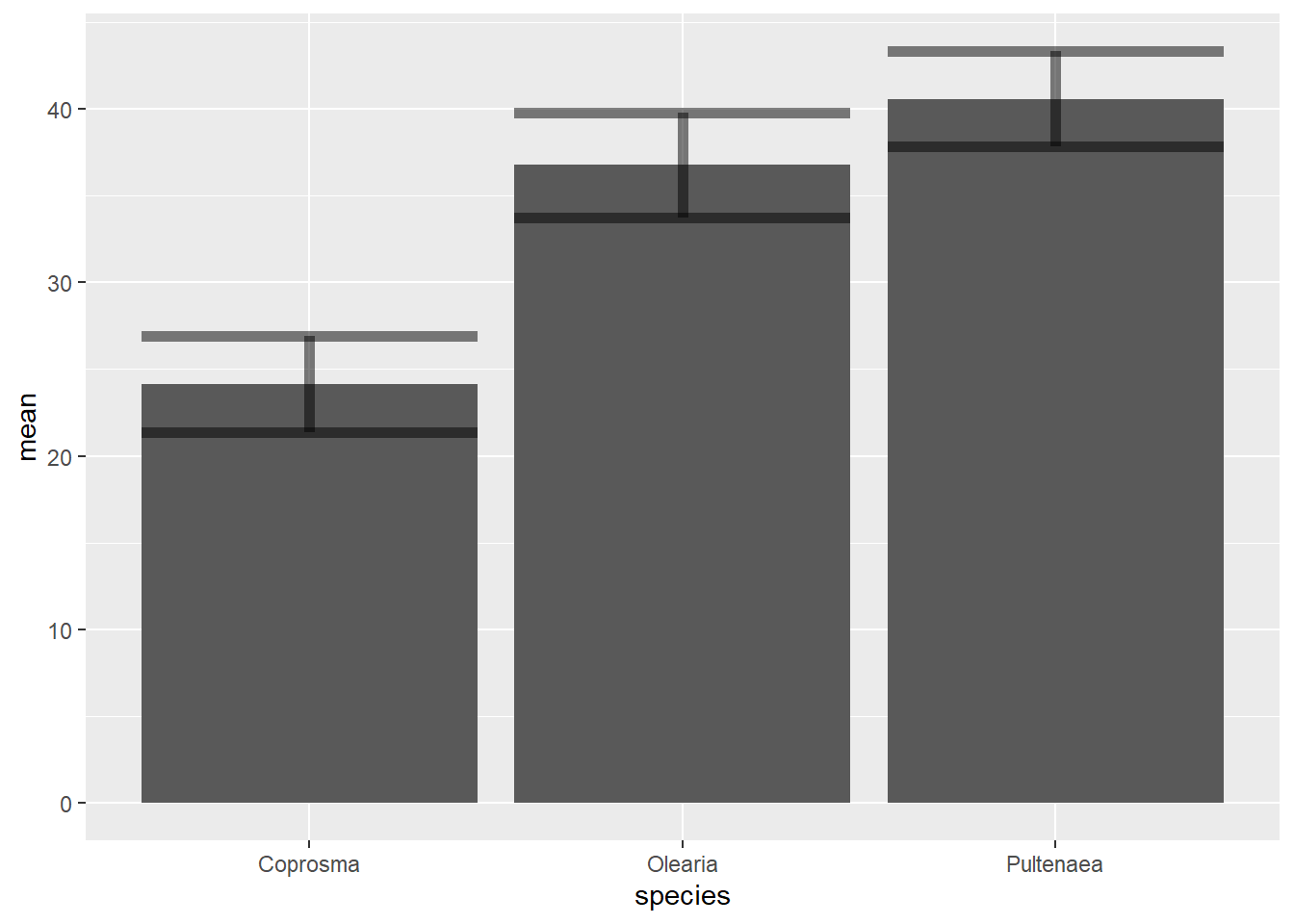#### Width

The width argument is arguably the most important aesthetical customisation for errorbars. Width customises the width of the errorbars compared to the width of the bars.

``````ggplot(weeds.summarise, aes(x=species, y=mean)) +
geom_bar(stat="identity")+
geom_errorbar(aes(ymin = mean-se, ymax = mean+se), width = 0.5)``````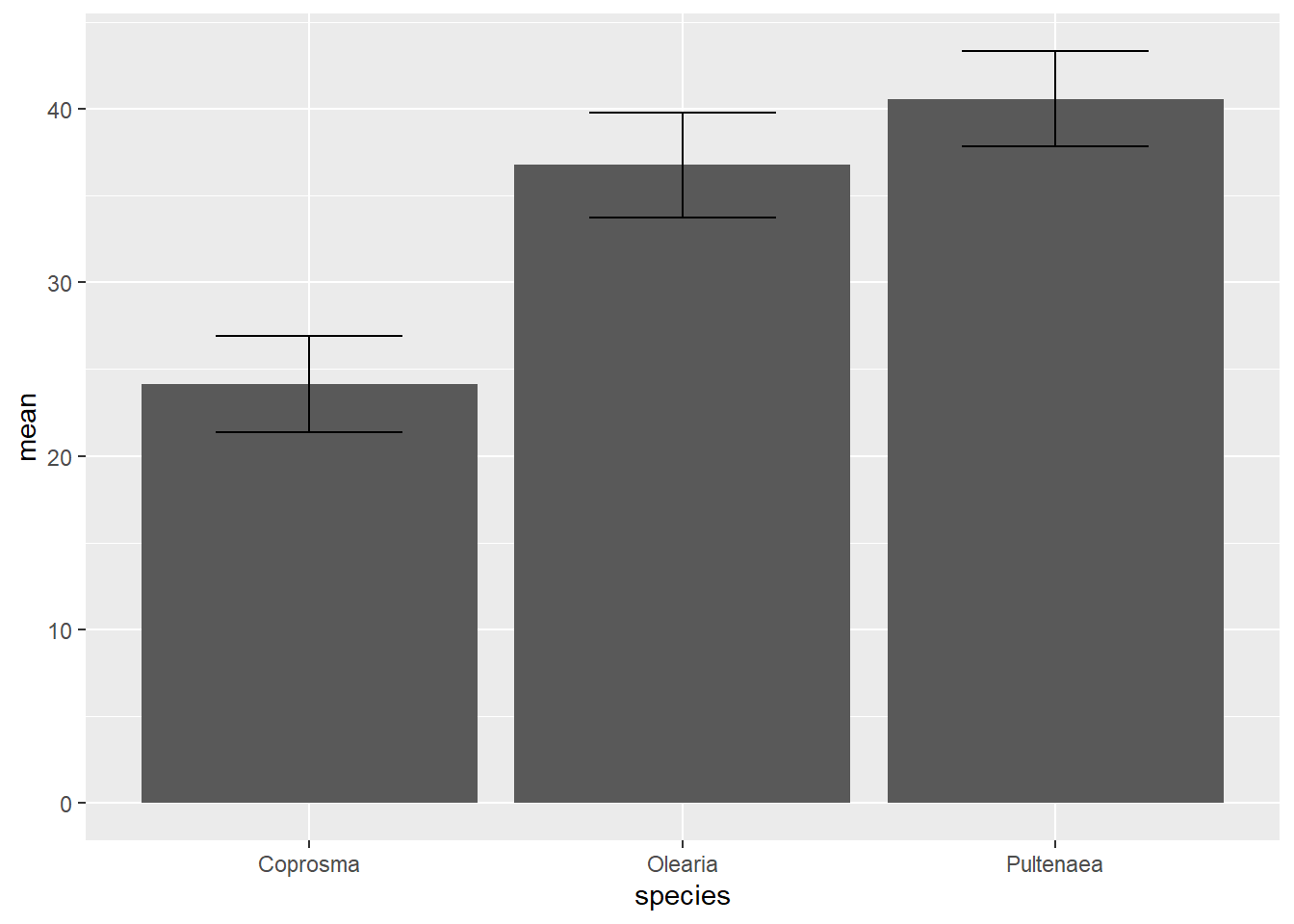The default width value for errorbars is 0.9, that is 90% of the width of the bar.